# Force & Its Types (बल एवम् इसके प्रकार)- Mechanics Physics

Physics topic – “Force & Its Types (बल एवम् इसके प्रकार)” is important for all competitive exams like CET- Common eligibility Test, SSC CGL, SSC CHSL, RRB NTPC, UPSC and other state civil services exams. In these exams, almost 4-5 questions are coming from Physics. Let’s start the topic – Mechanics Physics – Force & Its Types (बल एवम् इसके प्रकार)-

Force & Its Types.

(बल एवम् इस के प्रकार)

A force moves or tends to move the object, stops or tends to stop the motion of the object. The force can also change the direction of the motion of an object. It can also change the shape or size of a body on which it acts. It is a vector quantity it has magnitude as well as direction.

• The SI unit of force is Newton
• Denoted by N.

1 N = I kgms-2

Force acts differently according to the type of motion of the objects.

Types of forces:

• Centripetal force
• Centrifugal force
• Gravitational force.
• Cohesive force
• Electrostatic force
• Magnetic force
• Frictional force
• Tension force
•  Nuclear force.

During the curvilinear motion two types of force act: Centrifugal force and Centripetal force.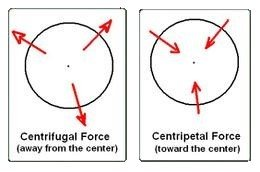Centripetal force (केन्द्राभिमुख बल):

• “The Centripetal force is the force acting towards the centre of the circular path.”
• This force is necessary to keep an object moving in a curved path and that is directed inward toward the centre of rotation.
• If a body of mass m moving on a circular path of radius r with uniform speed v, then the required centripetal force is: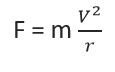Examples:

1) Planets orbiting around the Sun.

2) Revolving of electrons in their orbital.

3) Stone bind with thread and move with finger.

4) Rides in a roller coaster.

Centrifugal force (अभिकेन्द्रीय बल):

• “The centrifugal force acts in a direction pointing away from the centre of the circle.”
• This force is felt by an object moving in a curved path that acts outwardly away from the centre of rotation.
• Centrifugal force is equal in magnitude and opposite in direction to the centripetal force.
• Centrifugal force unit is Newton.

The centrifugal force drives the object away from the centre. So, centrifugal force can be calculated as: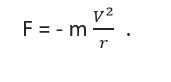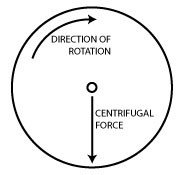Where,

is the Centrifugal force

m is the mass of the object

v   is the velocity or speed of the object.

Example of centrifugal force:

• Working of washing machine.
• Isolation of cream by crunching milk.
• Mud flying of a tire.

Gravitational force (गुरुत्वाकर्षण बल):

The gravitational force is a force that attracts any two objects with mass, then the force between them is known as Gravitational force.

The force of gravity is the force with which the earth, moon, or other massively large object attracts another object towards itself.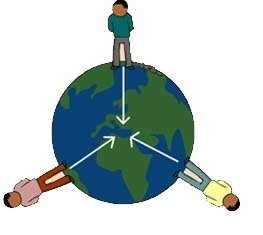• All objects in the universe pulls every other body with a force that is directly proportional to their mass-produced and inversely proportional to the distance square between them.

The force exerted by two bodies with mass m1 and m2, whose centres is r units apart is given as: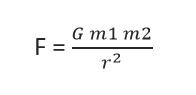Important facts:

(i) The “force of gravitational” occurs maximum at the poles of the earth, Mainly on the “North Pole”.

(ii) Gravitation force is “zero” at the centre of the earth.

(iii) Gravitation force is the weight of the object, Hence we can say that the weight of object maximum at the poles and zero at the centre of the earth.

• So, weight is directly proportional to gravitational force.

Weight   α   gravitational force

• It is a reason, the weight of any object on the earth become 1/6th at the moon.
• If a pendulum clock, placed on the surface of moon then, the period of oscillation increase and clock become slow.
• In pendulum clock, pendulum represent – second needle.

(iv) When we go above from the surface of the earth and towards the centre of earth. In both case, force of gravitational force become decrease.

• It is the reason, weight of free falling object become zero. And it is reason that astronauts feel weightless in space.

Cohesive force (एकजुट बल):

“The force of attraction between the same types of molecules” is known as cohesive forces.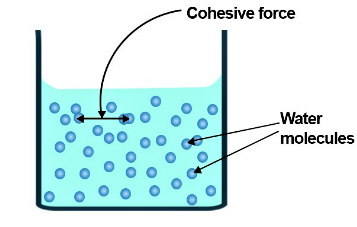• A strong force of cohesion forms water droplets on any surface.

Example: two drops of mercury become single when they come closer.

“The force of attraction between different types of molecules” is called adhesion force.

• In this force different type of molecule have tendency to bond with each other is known as Adhesion.
• A strong adhesion force causes the liquid to spread all over the surface.

Example of adhesion force:1) Glue and paper.

2) Cement and bricks.

3) Water between two glasses.

Electrostatics force (विद्युत बल):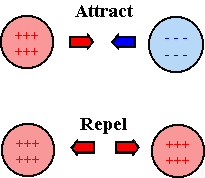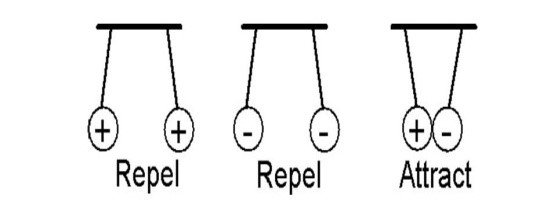• Like charges repel each other while unlike charges attract each other. If the charges are at rest then the force between them is known as the electrostatic force.
• The electrostatic force between charges increases when the magnitude of the charges increases or the distance between the charges decreases.
• The electrostatic force was first studied in detail by Charles- Augustin de Coulomb around 1784. So, it is also called Coulomb’s force.

the magnitude of the electrostatic force between two point-like charges is inversely proportional to the square of the distance between the charges: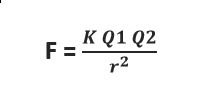Where

•     Q1 and Q2 are the magnitudes of the two charges.
•      r is the distance between the two charges.

Magnetic force (चुंबकीय बल) :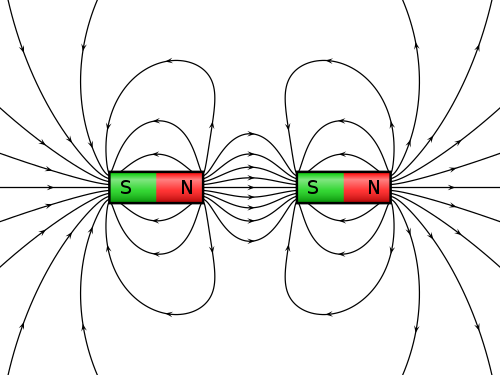• Magnetic Force can be defined as “the attractive or repulsive force that arises between the poles of a magnet and electrically charged moving particles because of their motion”.
• Hence, it is a consequence of the electromagnetic forces.
• It is the basic force responsible for such effects as the action of electric motors and the attraction of magnets for iron.

Frictional force (घर्षण बल):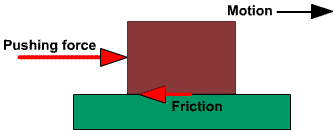• Frictional force comes from interactions with a surface as an object moves or tries to move relative to the surface.
• Friction results from the two surfaces being pressed together closely, causing inter-molecular attractive forces between molecules of different surfaces.

Tension force (तनाव बल):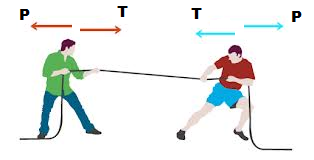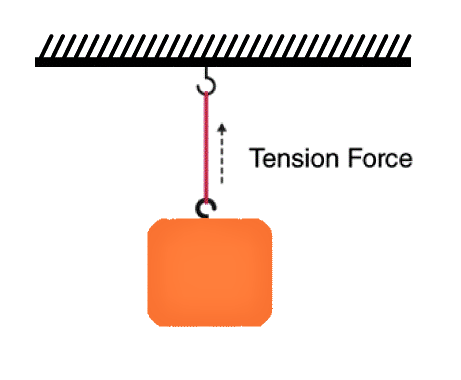• The tension force is the force that is transmitted through a string, rope, cable or wire when it is pulled tight by forces acting from opposite ends.
• The tension force is directed along the length of the wire and pulls equally on the objects on the opposite ends of the wire.

Nuclear Force (परमाणु बल):

###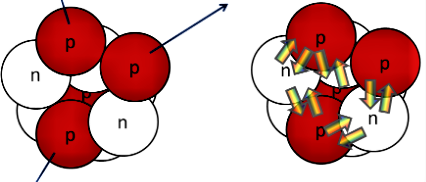• The nuclear force is the force that binds the protons and neutrons in a nucleus together. This force can exist between protons and protons, neutrons and protons or neutrons and neutrons.
• Hence, we can say that this force responsible for the bonding of nucleus. It is also known as nuclear interactions or strong forces.
• The nuclear force is about 10 millions times stronger than the chemical binding that holds atoms together in molecules.
• It is attractive in nature but with a repulsive core. That is the reason that the nucleus is held together without collapsing in itself.